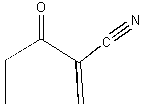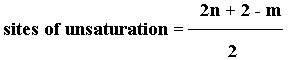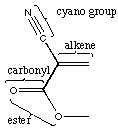Organic Chemistry Solutions: #19

19.* (1992 1 1) Consider the molecule shown below.A. How many sites of unsaturation does this molecule contain?

1 triple bond counts as two, there is 1 C=C bond and 1 C=O bond for a total of 4. Or you could use the formula below. Remember that n is the number of C's and m is the number of H's. You count halogens as H, ignore oxygen, and subtract 1 from m for each nitrogen.B. Is this substance chiral?

No. There is a plane of symmetry which passes through the entire molecule. That plane is the plane of the page.

C. Label the hybridization state of all of the carbon atoms in this molecule.D. Label the functional groups in this molecule.